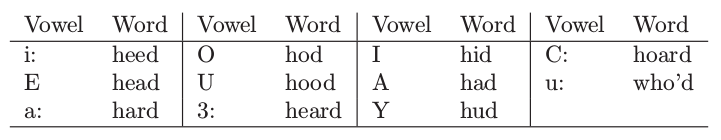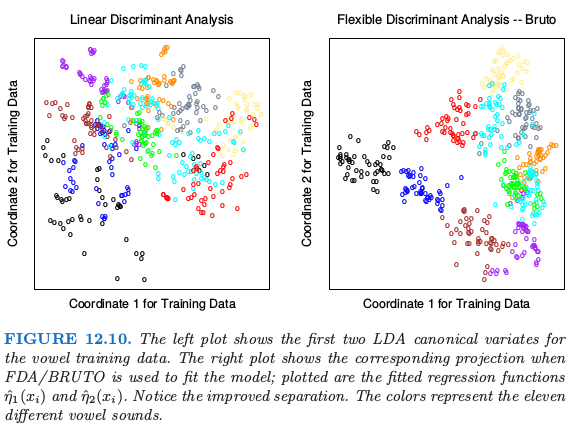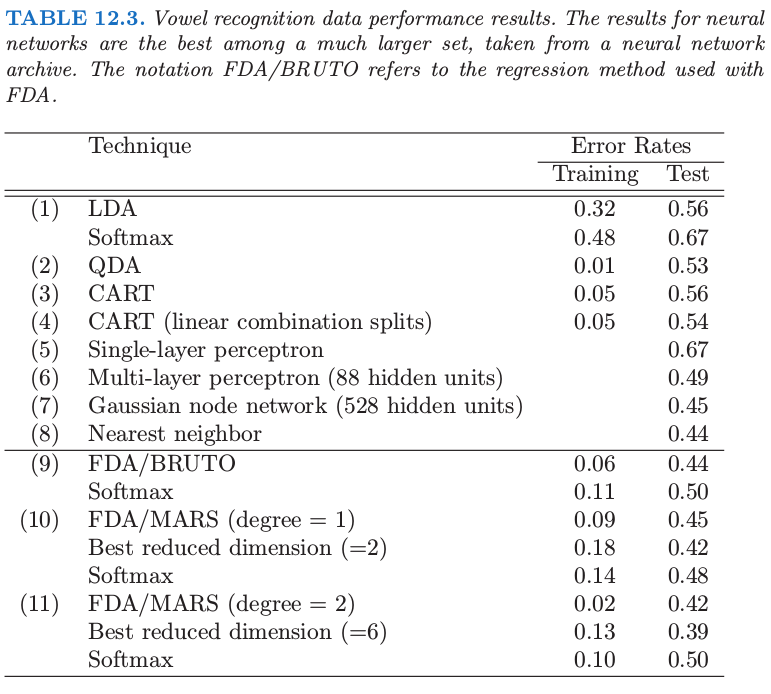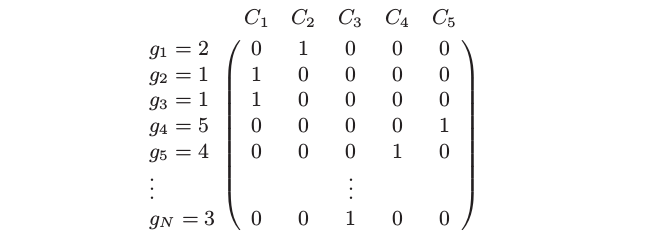12.5 FDA¶

4.3.2 节中，我们看到典则距离是高斯情形下分类所需要的全部条件，并且每个类别间有相等的协方差．总结下，就是：

LDA 可以通过一系列的线性回归来实现，将测试点划分到拟合空间中距离最近的那个类别重心的类中．这类似可以应用到降维后的版本，或者当 $L=K-1$ 时的全秩版本．FDA 模型采用自适应的可加样条回归函数来对 $\eta_\ell(x)$ 建模，并且在右图中的点有坐标 $\hat\eta_1(x_i)$ 和 $\hat\eta_2(x_i)$．在 S-PLUS 用的程序叫做 bruto，因此有了图的标题以及表格中的名称．我们看到这种情形下灵活模型帮助分离类别．表 12.3 展示了一系列分类技巧的训练误差和测试误差．FDA/MARS 指的是 Friedman 的多元自适应回归样条；degree = 2 表明允许成对交叉项．注意到对于 FDA/MARS，最优的分类记过是在降维后的子空间中得到的．计算 FDA 估计¶

FDA 坐标的计算在许多重要的情形中可以简化，特别是当非参回归过程可以表示成线性算子．我们将这种算子用 $\mathbf S_\lambda$ 表示，也就是 $\hat{\mathbf y}=\mathbf S_\lambda\mathbf y$，其中 $\mathbf y$ 是响应变量向量，而 $\hat {\mathbf y}$ 是拟合值的向量．如果固定光滑参数，可加样条有这样的性质，如果 MARS 一次性选出基函数，它也有这个性质．下标 $\lambda$ 表示整个光滑参数集．在这种情形中，最优得分等价于典则相关系数问题，并且解可以通过单特征值分解进行求解．这在练习 12.6 中有讨论，并且将得到的算法展现在这里．

weiya 注：Ex. 12.61. 多元非参回归 (Multivariate nonparametric regression)．在 $\mathbf X$ 上拟合 $\mathbf Y$ 的多响应、自适应的非参回归，得到拟合值 $\hat{\mathbf Y}$．令 $\mathbf S_\lambda$ 为拟合最终选择的模型的线性算子，并且 $\eta^*(x)$ 为拟合的回归函数的向量．
2. 最优得分 (Optimal scores)．计算 $\mathbf Y^T\hat{\mathbf Y}=\mathbf Y^T\mathbf S_\lambda\mathbf Y$ 的特征值分解，其中特征向量 $\mathbf \Theta$ 是标准化的：$\mathbf \Theta^T\mathbf D_\pi\mathbf \Theta = \mathbf I$．这里 $\mathbf D_\pi = \mathbf Y^T\mathbf Y/N$ 是类别先验概率的对角阵．
3. 更新 (Update)．采用最优得分 $\eta(x)=\mathbf\Theta^T\eta^*(x)$ 从第一步更新模型．

$\eta(x)$ 中 $K$ 个函数的第一个函数是常值函数——平凡解；剩下的 $K-1$ 个函数是判别函数．常值函数以及标准化使得所有剩下的函数都进行了中心化．

4.2 节我们讨论了在指示响应变量矩阵上使用回归用作分类的方法的缺点．具体地，会出现三个或更多类别的 masking 问题．FDA 在第 (1) 步中也用了这样的回归的拟合，但是然后进一步转换得到有用的判别函数，因此它们没有这些缺点．练习 12.9 从另外一个角度解释了这个现象．

weiya 注：Ex. 12.9